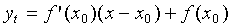# Tangent To The Curve

Hi guys, i'm having a little trouble finding the equation of the tangent to the curve 2e^(xy) - ysinx = log(y) + 2 at the point (0,1).
I've basically concluded that either the equationshould be used or either find the gradient at (0,1) through differentiation and then use (y-y1)=m(x-x1).
But i can't seem to get the derivative in terms of y. Any help or advice would be much appreciated, thank you.

vsage
I think the point was that you can't get the equation in terms of a solvable variable. You can, however, probably implicitly differentiate the above equation with respect to y and with respect to x. Have you tried that path yet?

HallsofIvy